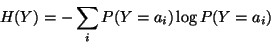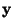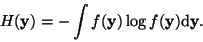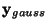Next: Approximations of negentropy Up: Measures of nongaussianity Previous: Kurtosis

### Negentropy

A second very important measure of nongaussianity is given by negentropy. Negentropy is based on the information-theoretic quantity of (differential) entropy.

Entropy is the basic concept of information theory. The entropy of a random variable can be interpreted as the degree of information that the observation of the variable gives. The more random'', i.e. unpredictable and unstructured the variable is, the larger its entropy. More rigorously, entropy is closely related to the coding length of the random variable, in fact, under some simplifying assumptions, entropy is the coding length of the random variable. For introductions on information theory, see e.g. [8,36].

Entropy H is defined for a discrete random variable Y as(17)

where the ai are the possible values of Y. This very well-known definition can be generalized for continuous-valued random variables and vectors, in which case it is often called differential entropy. The differential entropy Hof a random vectorwith densityis defined as [8,36]:(18)

A fundamental result of information theory is that a gaussian variable has the largest entropy among all random variables of equal variance. For a proof, see e.g. [8,36]. This means that entropy could be used as a measure of nongaussianity. In fact, this shows that the gaussian distribution is the most random'' or the least structured of all distributions. Entropy is small for distributions that are clearly concentrated on certain values, i.e., when the variable is clearly clustered, or has a pdf that is very spiky''.

To obtain a measure of nongaussianity that is zero for a gaussian variable and always nonnegative, one often uses a slightly modified version of the definition of differential entropy, called negentropy. Negentropy J is defined as follows(19)

whereis a Gaussian random variable of the same covariance matrix as. Due to the above-mentioned properties, negentropy is always non-negative, and it is zero if and only ifhas a Gaussian distribution. Negentropy has the additional interesting property that it is invariant for invertible linear transformations [7,23].

The advantage of using negentropy, or, equivalently, differential entropy, as a measure of nongaussianity is that it is well justified by statistical theory. In fact, negentropy is in some sense the optimal estimator of nongaussianity, as far as statistical properties are concerned. The problem in using negentropy is, however, that it is computationally very difficult. Estimating negentropy using the definition would require an estimate (possibly nonparametric) of the pdf. Therefore, simpler approximations of negentropy are very useful, as will be discussed next.Next: Approximations of negentropy Up: Measures of nongaussianity Previous: Kurtosis
Aapo Hyvarinen
2000-04-19